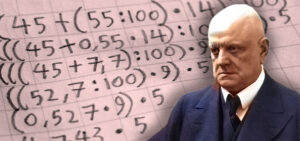# Sibeliplus and minus (New Year Quiz 2021) – SolutionsOur 2021 New Year Quiz is a series of mathematical riddles related to Sibelius and his music. In each case the correct answer is a piece of music by Sibelius: match the number you obtain from each calculation with Sibelius’s opus number list to find the titles. The answers are listed below:

(1) The number (total!) of daughters of Jean and Aino Sibelius PLUS the number of times Sibelius visited England. Answer: Karelia Suite (6+5=11)

(2) The number of completed string quartets by Sibelius TIMES the number of movements in the King Christian II suite (standard, published version). Answer: Malinconia (4×5=20)

(3) The number of pieces in Sibelius’s Op. 76 TIMES the number of pieces in Sibelius’s Op. 77. Answer: Finlandia (13×2=26)

(4) The number of movements in Sibelius’s Piano Quintet PLUS the number of movements in his ‘Hafträsk’ Trio. Answer: En saga (5+4=9)

(5) The number of symphonies Sibelius wrote TIMES the number of musical numbers in the original theatre music for Kuolema. Answer: Romance in C major for strings (7×6=42)

(6) The number of years Sibelius lived at Ainola MINUS the number of times he visited the USA. Answer: Symphony No. 3 (53–1=52)

(7) The highest number in Sibelius’s official opus number list DIVIDED BY the number of movements in his Sixth Symphony. Answer: Snöfrid (116/4=29)

(8) The number of strophes usually sung in the Finlandia Hymn PLUS the number of surviving alternative versions of Rakastava. Answer: Kullervo (2+5=7)

(9) ADD the number of trees in Op. 75 to the number of flowers in Op. 85 and then MULTIPLY the total by the number of flowers in Op. 88. Answer: Two Songs from ‘Twelfth Night’ ([5+5]x6=60)

(10) MULTIPLY the number of trombones needed in the Second Symphony by the number of horns in the Fourth Symphony and then ADD the number of trumpets in the Seventh Symphony. Answer: The Wood-Nymph ([3×4]+3=15)

We hope you enjoyed this quiz! Try previous year’s quizzes by clicking here.

Image credits:
Background: © 2018 Santeri Viinamäki (Creative Commons Attribution-Share Alike 4.0, cropped and tinted)
Sibelius: Pietinen, 1935 / Museovirasto, CC BY 4.0